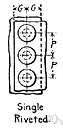# unary

Also found in: Thesaurus, Encyclopedia, Wikipedia.
Related to unary: Unary operator

## unary

(ˈjuːnərɪ)
consisting of, or affecting, a single element or component; monadic
[C16 (in the obsolete sense: a unit): from Latin unus one + -ary]
ThesaurusAntonymsRelated WordsSynonymsLegend:
 Adj. 1unary - consisting of or involving a single element or component; "in a unary operation in a mathematical system one element is used to yield a single result"single - existing alone or consisting of one entity or part or aspect or individual; "upon the hill stood a single tower"; "had but a single thought which was to escape"; "a single survivor"; "a single serving"; "a single lens"; "a single thickness"
Mentioned in ?
References in periodicals archive ?
The GR code starts with 18 leading zeros that are equivalent to the unary representation of the quotient.
To assess the performance of algorithms in this experiment, three quality indicators are considered: Additive Unary [epsilon]-indicator ([I.sup.1.sub.[epsilon]+]) , hypervolume ([I.sub.HV]) , and the Inverted Generational Distance (IGD) .
Another tree parameter that leads to the same type of distribution is the longest chain of unary nodes in a unary-binary tree, which was studied in [8,25].
Then the code word calculated would be converted into unary code.
where z is the partition function, [[psi].sub.i] ([x.sub.i],Y) and [[psi].sub.ij] ([x.sub.i], [x.sub.j], Y) are unary and pairwise potentials, respectively, and [N.sub.i] denotes the neighbours of pixel i.
EquiKeep ([chi]) is a unary algebraic operator that as the Selection([sigma]) operator, evaluates a logical expression from a relation R, but EquiKeep applies the logical expression to the columns (attributes) of R.
There are three main options for correction: the first is a flat correction (A'-B'-C in the chart); the second is a common unary zigzag (A'-B'-C'); the third is a double zigzag (A'-B'-C'-X-Aa-Ba-Ca).
The segmentation information was merely integrated into unary terms or pairwise terms rather than higher order terms.
An involution poset (IP) is a poset (X, [less than or equal to], c) with a unary operation c: x [member of] X [right arrow] [x.sup.c] [member of] X, such that
One of those examples the problem of unary addition--was selected as a suitable sample for processing within the analysis.
However, the population was split quite evenly between L and B, with a small minority of Bits who still remembered the single-tape Turing machine and preferred unary encoding (U), without any of this endianness business.
Let Q be a unital quantale, [perpendicular] : Q [right arrow] Q an unary operation on Q.

Site: Follow: Share:
Open / Close